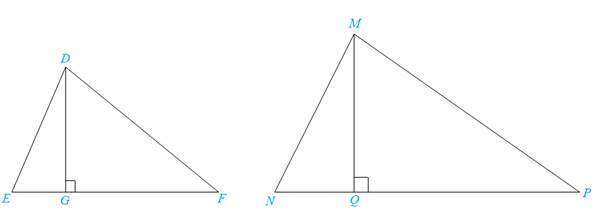Chapter 5.3, Problem 35EElementary Geometry For College St...

7th Edition
Alexander + 2 others
ISBN: 9781337614085

Solutions

Chapter
SectionElementary Geometry For College St...

7th Edition
Alexander + 2 others
ISBN: 9781337614085
Textbook Problem

Use a two-column proof to prove the following theorem:“The lengths of the corresponding altitudes of similar triangles have the same ratio as lengths of any pair of corresponding sides.”Given:                           Δ D E F ∼ Δ M N P ; D G ¯ and M Q ¯ are altitudesProve:                             D G M Q = D E M NTo determine

To prove:

The theorem “The lengths of the corresponding altitudes of similar triangles have the same ratio as lengths of any pair of corresponding sides.” By using two-column proof and prove DGMQ=DEMN by using given information.

Explanation

Definition:

AA:

If two angles of one triangle are congruent to two angles of another triangle, then the triangles are similar.

CSSTP:

Corresponding sides of similar triangles are proportional.

CASTC:

Corresponding angles of similar triangles are congruent.

Description:

Given that ΔDEFΔMNP, DG¯ and MQ¯ are altitudes.

The given figure is shown below.

Figure

From the given figure, it is observed that DG¯ and MQ¯ are altitudes.

It is known that an altitude is a line segment through a vertex and perpendicular to a line of base. That is, DG¯EF¯ and MQ¯NP¯.

Since, perpendicular lines form a right angle triangle, DGE and MQN are right angle triangles.

Right angle triangles are congruent. So that DGE=MQN.

From the above mentioned CASTC definition, EN since corresponding angles of similar triangles are congruent.

The above mentioned AA definition, the two triangles DGE and MQN are similar since the two angles of one triangle are congruent to two angles of another triangle. Hence, ΔDGEΔMQN.

From the definition of CSSTP, corresponding sides of similar triangles are proportional.

That is, DGMQ=DEMN

Still sussing out bartleby?

Check out a sample textbook solution.

See a sample solution

The Solution to Your Study Problems

Bartleby provides explanations to thousands of textbook problems written by our experts, many with advanced degrees!

Get Started

Find the value of the sum. 21. i=48(3i2)

Single Variable Calculus: Early Transcendentals, Volume I

23-42 Find f. f(x)=x(6+5x),f(1)=10

Calculus (MindTap Course List)

In Exercises 4756, solve the given equation for the indicated variable. 27=32x1

Finite Mathematics and Applied Calculus (MindTap Course List)

Solve each inequality. 02x+342

Trigonometry (MindTap Course List)

True or False: Predator-prey population models are the solutions to two differential equations.

Study Guide for Stewart's Single Variable Calculus: Early Transcendentals, 8th